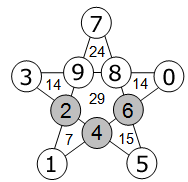Select Page

The number within the five triangles of the star is equal to the sum of the three numbers in its surrounding circles.  The number in the center of the star is equal to the sum of the five numbers in its surrounding circles. The numbers in the circles can only be 0 to 9 and each number can be used only once.  Three numbers have been provided to get you started.  Find the remaining seven numbers.[donotprint]

### Solution### Solution explanationComments on solving the puzzle; a process of eliminating possible values as answers:

Values 2, 4, 6 are provided and can not be reused.

Starting at Equations (1a & 1b), unknowns F & G are equal to 1 or 5 respectively as shown.

Equation (2): unknowns D & E can only be equal to 0 or 8 as shown. All other combinations include either a 1, 2, 4, 5 or 6 value.

Equation (3): unknowns A, C & D can be equal to 7, 8 or 9 only as shown. Comparing with Equation (2), D can only be equal to 8 and then E is equal to 0. A & C are then equal to 7 or 9 as shown.

Equation (4): unknowns B & C can be equal to 3 or 9 as shown. Comparing with Equation (3), C can only be equal 9 and A is equal to 7 and B is then equal to 3.

Equation (5): 9 + 8 + 6 + 4 + 2 = 29 and thus verifies the center sum of 29.

Now insert your final answers into the original puzzle and verify the resulting sums.

## Feedback

There are more than one way of doing these puzzles and may well be more than one answer.  Please let me and others know what alternatives you find by commenting below.  We also welcome general comments on the subject and any feedback you’d like to give.   If you have a question that need a response from me or you would like to contact me privately, please use the contact form.[/donotprint]

## Get more puzzles!

If you’ve enjoyed doing the puzzles, consider ordering the book; 150+ of the best puzzles in a handy pocket sized format. [donotprint]Click here for full details.[/donotprint]

### Share This

Share this post with your friends!